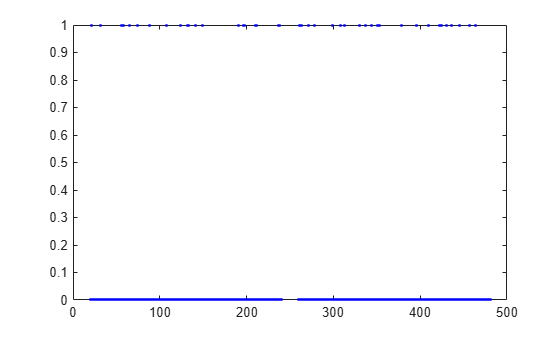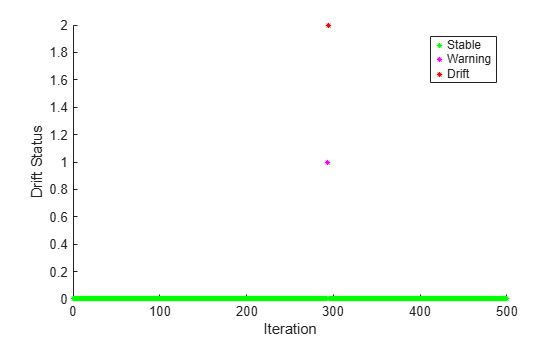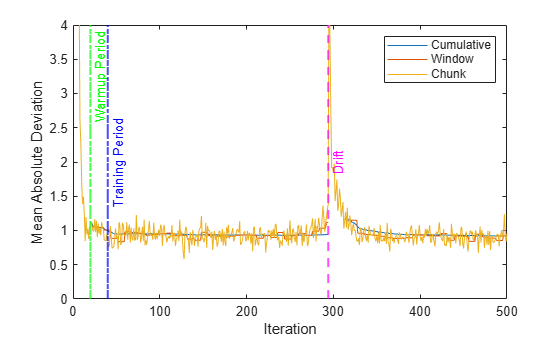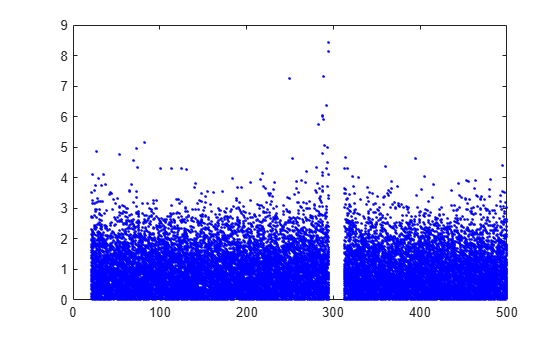# loss

Regression or classification error of incremental drift-aware learner

## Syntax

``Err = loss(Mdl,X,Y)``
``Err = loss(Mdl,X,Y,Name=Value)``

## Description

example

````Err = loss(Mdl,X,Y)` returns the regression or classification error for model `Mdl` trained using predictors in `X` and true observed values in `Y`.`Err` is an n-by-1 vector, where n is the number of observations.```

example

````Err = loss(Mdl,X,Y,Name=Value)` specifies additional options using one or more name-value arguments. For example, you can specify the dimension of the predictor data and the loss function to compute.```

## Examples

collapse all

Load the human activity dataset. Randomly shuffle the data.

```load humanactivity; n = numel(actid); rng(123) % For reproducibility idx = randsample(n,n);```

For details on the data set, enter `Description` at the command line.

Define the predictor and response variables.

```X = feat(idx,:); Y = actid(idx);```

Responses can be one of five classes: Sitting, Standing, Walking, Running, or Dancing.

Dichotomize the response by identifying whether the subject is moving (actid > 2).

`Y = Y > 2;`

Flip labels for the second half of the dataset to simulate drift.

`Y(floor(numel(Y)/2):end,:) = ~Y(floor(numel(Y)/2):end,:);`

Initiate a default incremental drift-aware model for classification as follows:

1. Create a default incremental linear SVM model for binary classification.

2. Initiate a default incremental drift-aware model using the incremental linear SVM model as the base learner.

```BaseLearner = incrementalClassificationLinear(); idaMdl = incrementalDriftAwareLearner(BaseLearner);```

`idaMdl` is an `incrementalDriftAwareLearner` model. All its properties are read-only.

Preallocate the number of variables in each chunk for creating a stream of data and the variable to store the classification error.

```numObsPerChunk = 50; nchunk = floor(n/numObsPerChunk); ce = array2table(zeros(nchunk,3),VariableNames=["Cumulative" "Window" "Loss"]); PoL = zeros(nchunk,numObsPerChunk); % To store per observation loss values driftTimes = [];```

Simulate a data stream with incoming chunks of 50 observations each. At each iteration:

1. Call `updateMetrics` to measure the cumulative performance and the performance within a window of observations. Overwrite the previous incremental model with a new one to track performance metrics.

2. Call `fit` to fit the model to the incoming chunk. Overwrite the previous incremental model with a new one fitted to the incoming observations.

3. Call `perObservationLoss` to compute classification error on each observation in the incoming chunk of data.

4. Call `loss` to measure the model performance on the incoming chunk.

5. Store all performance metrics in `ce` to see how they evolve during incremental learning. The `Metrics` property of `idaMdl` stores the cumulative and window classification error, which is updated at each iteration. Store the loss values for each chunk in the third column of `ce`.

```for j = 1:nchunk ibegin = min(n,numObsPerChunk*(j-1)+1); iend = min(n,numObsPerChunk*j); idx = ibegin:iend; idaMdl = updateMetrics(idaMdl,X(idx,:),Y(idx)); idaMdl = fit(idaMdl,X(idx,:),Y(idx)); PoL(j,:) = perObservationLoss(idaMdl,X(idx,:),Y(idx)); ce{j,["Cumulative" "Window"]} = idaMdl.Metrics{"ClassificationError",:}; ce{j,"Loss"} = loss(idaMdl,X(idx,:),Y(idx)); if idaMdl.DriftDetected driftTimes(end+1) = j; end end```

The `updateMetrics` function evaluates the performance of the model as it processes incoming observations. The function writes specified metrics, measured cumulatively and within a specified window of processed observations, to the `Metrics` model property. The fit function fits the model by updating the base learner and monitoring for drift given an incoming batch of data.

Plot the cumulative and per window classification error. Mark the warmup and training periods, and where the drift was introduced.

```h = plot(ce.Variables); xlim([0 nchunk]) ylim([0 0.07]) ylabel("Classification Error") xlabel("Iteration") xline(idaMdl.MetricsWarmupPeriod/numObsPerChunk,"g-.","Warmup Period",LineWidth= 1.5) xline(idaMdl.TrainingPeriod/numObsPerChunk,"b-.","Training Period",LabelVerticalAlignment="middle",LineWidth= 1.5) %xline(floor(numel(Y)/2)/numObsPerChunk,"m--","Drift",LabelVerticalAlignment="middle",LineWidth= 1.5) xline(driftTimes,"m--","Drift",LabelVerticalAlignment="middle",LineWidth=1.5) legend(h,ce.Properties.VariableNames) legend(h,Location="best")```The yellow line represents the classification error on each incoming chunk of data. `loss` is agnostic of the metrics warm-up period, so it measures the classification error for all iterations. After the metrics warm-up period, `idaMdl` tracks the cumulative and window metrics.

Plot the per observation loss.

```figure() plot(PoL,'b.');````perObservationLoss` computes the classification loss for each observation in the incoming chunk of data.

Create the random concept data and the concept drift generator using the helper functions `HelperRegrGenerator` and `HelperConceptDriftGenerator`, respectively.

```concept1 = HelperRegrGenerator(NumFeatures=100,NonZeroFeatures=[1,20,40,50,55], ... FeatureCoefficients=[4,5,10,-2,-6],NoiseStd=1.1); concept2 = HelperRegrGenerator(NumFeatures=100,NonZeroFeatures=[1,20,40,50,55], ... FeatureCoefficients=[4,7,10,-1,-5],NoiseStd=1.1); driftGenerator = HelperConceptDriftGenerator(concept1,concept2,15000,1250);```

`HelperRegrGenerator` generates streaming data using features and feature coefficients for regression specified in the call to the function. At each step, the function samples the predictors from a normal distribution. Then, it computes the response using the feature coefficients and predictor values and adding a random noise from a normal distribution with mean zero and specified noise standard deviation.

`HelperConceptDriftGenerator` establishes the concept drift. The object uses a sigmoid function `1./(1+exp(-4*(numobservations-position)./width))` to decide the probability of choosing the first stream when generating data . In this case, the position argument is 15000 and the width argument is 1250. As the number of observations exceeds the position value minus half of the width, the probability of sampling from the first stream when generating data decreases. The sigmoid function allows a smooth transition from one stream to the other. Larger width values indicate a larger transition period where both streams are approximately equally likely to be selected.

Configure an incremental drift-aware model for regression as follows:

1. Create an incremental linear model for regression: Track the mean absolute deviation (MAD) to measure the performance of the model. Create an anonymous function that measures the absolute error of each new observation. Create a structure array containing the name `MeanAbsoluteError` and its corresponding function. Specify a metrics warm-up period of 1000 observations. Specify a metrics window size of 500 observations.

2. Initiate an incremental concept drift detector for continuous data. Use the Hoeffding's Bounds Drift Detection Method with moving average (HDDMA).

3. Using the incremental linear model and the concept drift detector, instantiate an incremental drift-aware model. Specify the training period as 1000 observations.

```maefcn = @(z,zfit,w)(abs(z - zfit)); % Mean absolute deviation function maemetric = struct(MeanAbsoluteError=maefcn); baseMdl = incrementalRegressionLinear(MetricsWarmupPeriod=1000,MetricsWindowSize=400,Metrics=maemetric,EstimationPeriod=0); dd = incrementalConceptDriftDetector("hddma",Alternative="greater",InputType="continuous"); idaMdl = incrementalDriftAwareLearner(baseMdl,DriftDetector=dd,TrainingPeriod=2000);```

Generate an initial sample of 20 observations and configure the model to predict responses by fitting it to the initial sample.

```initobs = 20; rng(1234); % For reproducibility [driftGenerator,X,Y] = hgenerate(driftGenerator,initobs); idaMdl = fit(idaMdl,X,Y);```

Preallocate the number of variables in each chunk and number of iterations for creating a stream of data, the variables to store the classification error, drift status, and drift time(s).

```numObsPerChunk = 50; numIterations = 500; mae = array2table(zeros(numIterations,3),VariableNames=["Cumulative" "Window" "Chunk"]); PoL = zeros(numIterations,numObsPerChunk); % Per observation loss values driftTimes = []; dstatus = zeros(numIterations,1); statusname = strings(numIterations,1);```

Simulate a data stream with incoming chunks of 50 observations each and perform incremental drift-aware learning. At each iteration:

1. Simulate predictor data and labels, and update the drift generator using the helper function `hgenerate`.

2. Call `updateMetrics` to compute cumulative and window metrics on the incoming chunk of data. Overwrite the previous incremental model with a new one fitted to overwrite the previous metrics.

3. Call `loss` to compute the MAD on the incoming chunk of data. Whereas the cumulative and window metrics require that custom losses return the loss for each observation, `loss` requires the loss on the entire chunk. Compute the mean of the absolute deviation.

4. Call `perObservationLoss` to compute the per observation regression error.

5. Call `fit` to fit the incremental model to the incoming chunk of data.

6. Store the cumulative, window, and chunk metrics and per observation loss to see how they evolve during incremental learning.

```for j = 1:numIterations % Generate data [driftGenerator,X,Y] = hgenerate(driftGenerator,numObsPerChunk); % Perform incremental fitting and store performance metrics idaMdl = updateMetrics(idaMdl,X,Y); PoL(j,:) = perObservationLoss(idaMdl,X,Y,'LossFun',@(x,y,w)(maefcn(x,y))); mae{j,1:2} = idaMdl.Metrics{"MeanAbsoluteError",:}; mae{j,3} = loss(idaMdl,X,Y,LossFun=@(x,y,w)mean(maefcn(x,y,w))); idaMdl = fit(idaMdl,X,Y); statusname(j) = string(idaMdl.DriftStatus); if idaMdl.DriftDetected driftTimes(end+1) = j; dstatus(j) = 2; elseif idaMdl.WarningDetected dstatus(j) = 1; else dstatus(j) = 0; end end```

`idaMdl` is an `incrementalDriftAwareLearner` model object trained on all the data in the stream. During incremental learning and after the model is warm, `updateMetrics` checks the performance of the model on the incoming observations, and the `fit` function fits the model to those observations.

Plot the drift status.

```gscatter(1:numIterations,dstatus,statusname,'gmr','*',4,'on',"Iteration","Drift Status") xlim([0 numIterations])```Plot the performance metrics to see how they evolved during incremental learning.

```figure h = plot(mae.Variables); xlim([0 numIterations]) ylim([0 4]) ylabel("Mean Absolute Deviation") xlabel("Iteration") xline(idaMdl.MetricsWarmupPeriod/numObsPerChunk,"g-.","Warmup Period",LineWidth= 1.5) xline(idaMdl.TrainingPeriod/numObsPerChunk,"b-.","Training Period",LabelVerticalAlignment="middle",LineWidth= 1.5) xline(driftTimes,"m--","Drift",LabelVerticalAlignment="middle",LineWidth=1.5) legend(h,mae.Properties.VariableNames)```The plot suggests the following:

• `updateMetrics` computes the performance metrics after the metrics warm-up period only.

• `updateMetrics` computes the cumulative metrics during each iteration.

• `updateMetrics` computes the window metrics after processing 400 observations

• Because `idaMdl` was configured to predict observations from the beginning of incremental learning, `loss` can compute the MAD on each incoming chunk of data.

Plot the per observation loss.

```figure() plot(PoL,'b.');````perObservationLoss` computes the classification loss for each observation in the incoming chunk of data after the metrics warm-up period.

## Input Arguments

collapse all

Incremental drift-aware learning model fit to streaming data, specified as an `incrementalDriftAwareLearner` model object. You can create `Mdl` using the `incrementalDriftAwareLearner` function. For more details, see the object reference page.

Batch of predictor data with which to compute loss, specified as a floating-point matrix of n observations and `Mdl.BaseLearner.NumPredictors` predictor variables.

When `Mdl.BaseLearner` accepts the `ObservationsIn` name-value argument, the value of `ObservationsIn` determines the orientation of the variables and observations. The default `ObservationsIn` value is `"rows"`, which indicates that observations in the predictor data are oriented along the rows of `X`.

The length of the observation responses (or labels) `Y` and the number of observations in `X` must be equal; `Y(j)` is the response (or label) of observation j (row or column) in `X`.

Note

`loss` supports only floating-point input predictor data. If your input data includes categorical data, you must prepare an encoded version of the categorical data. Use `dummyvar` to convert each categorical variable to a numeric matrix of dummy variables. Then, concatenate all dummy variable matrices and any other numeric predictors. For more details, see Dummy Variables.

Data Types: `single` | `double`

Chunk of responses or labels with which to compute loss, specified as one of the following.

• Floating-point vector of n elements for regression models, where n is the number of rows in `X`..

• Categorical, character, or string array, logical vector, or cell array of character vectors for classification models. If `Y` is a character array, it must have one class label per row. Otherwise, it must be a vector with n elements.

The length of `Y` and the number of observations in `X` must be equal; `Y(j)` is the response (or label) of observation j (row or column) in `X`.

For classification problems:

• If `Y` contains a label that is not a member of `Mdl.BaseLearner.ClassNames`, `loss` issues an error.

• The data type of `Y` and `Mdl.BaseLearner.ClassNames` must be the same.

Data Types: `single` | `double` | `categorical` | `char` | `string` | `logical` | `cell`

### Name-Value Arguments

Specify optional pairs of arguments as `Name1=Value1,...,NameN=ValueN`, where `Name` is the argument name and `Value` is the corresponding value. Name-value arguments must appear after other arguments, but the order of the pairs does not matter.

Example: `ObservationsIn="columns",Weights=W` specifies that the columns of the predictor matrix correspond to observations, and the vector `W` contains observation weights to apply.

Loss function, specified as a built-in loss function name or function handle.

The following table lists the built-in loss function names.

• For Regression Models:

NameDescription
`"mse"`Mean squared error
`"epsiloninsensitive"`Epsilon insensitive error

Default is `"mse"` for regression models.

• For Classification Models:

NameDescription
`"binodeviance"`Binomial deviance
`"classiferror"`Misclassification error rate
`"exponential"`Exponential
`"hinge"`Hinge
`"logit"`Logistic
`"quadratic"`Quadratic
`"mincost"`Minimal expected misclassification cost (for `incrementalClassificationNaiveBayes` only)

Default is `"mincost"` for `incrementalClassificationNaiveBayes` model object and `"classiferror"` for other classification objects.

Note

You can only specify `"classiferror"` for `incrementalClassificationECOC`.

To specify a custom loss function, use function handle notation. The function must have one of these forms:

• For Regression Models:

`lossval = lossfcn(Y,YFit,W)`

• The output argument `lossval` is a floating-point scalar.

• You specify the function name (`lossfcn`).

• `Y` is a length n numeric vector of observed responses.

• `YFit` is a length n numeric vector of corresponding predicted responses.

• `W` is a length n numeric vector of observation weights.

• For Classification Models:

`lossval = lossfcn(C,S,W)`

• The output argument `lossval` is an n-by-1 floating-point vector, where n is the number of observations in `X`. The value in `lossval(j)` is the classification loss of observation `j`.

• You specify the function name (`lossfcn`).

• `C` is an n-by-K logical matrix with rows indicating the class to which the corresponding observation belongs. `K` is the number of distinct classes (`numel(Mdl.BaseLearner.ClassNames)`), and the column order corresponds to the class order in the `Mdl.BaseLearner.ClassNames` property. Create `C` by setting `C(p,q)` = `1`, if observation `p` is in class `q`, for each observation in the specified data. Set the other elements in row `p` to `0`.

• `S` is an n-by-K numeric matrix of predicted classification scores. `S` is similar to the `Score` output of `predict`, where rows correspond to observations in the data and the column order corresponds to the class order in the `Mdl.BaseLearner.ClassNames` property. `S(p,q)` is the classification score of observation `p` being classified in class `q`.

• `W` is a length n numeric vector of observation weights.

Example: `LossFun="logit"`

Example: `LossFun=@lossfcn`

Data Types: `char` | `string` | `function_handle`

Predictor data observation dimension, specified as `"columns"` or `"rows"`.

`loss` supports `ObservationsIn` only if `Mdl.BaseLearner` supports the `ObservationsIn` name-value argument.

Example: `ObservationsIn="columns"`

Data Types: `char` | `string`

Chunk of observation weights, specified as a floating-point vector of positive values. `loss` weighs the observations in `X` with the corresponding values in `Weights`. The size of `Weights` must equal n, which is the number of observations in `X`.

By default, `Weights` is `ones(n,1)`.

Example: `Weights=w`

Data Types: `double` | `single`

 Barros, Roberto S.M. , et al. "RDDM: Reactive drift detection method." Expert Systems with Applications. vol. 90, Dec. 2017, pp. 344-55. https://doi.org/10.1016/j.eswa.2017.08.023

 Bifet, Albert, et al. "New Ensemble Methods for Evolving Data Streams." Proceedings of the 15th ACM SIGKDD International Conference on Knowledge Discovery and Data Mining. ACM Press, 2009, p. 139. https://doi.org/10.1145/1557019.1557041.

 Gama, João, et al. "Learning with drift detection". Advances in Artificial Intelligence – SBIA 2004, edited by Ana L. C. Bazzan and Sofiane Labidi, vol. 3171, Springer Berlin Heidelberg, 2004, pp. 286–95. https://doi.org/10.1007/978-3-540-28645-5_29.